Warning: Illegal string offset 'html' in /home/hsn/public_html/forum/cache/skin_cache/cacheid_1/skin_topic.php on line 909

1 = 2 Proved - HSN forum - Page 2# 1 = 2 Proved

54 replies to this topic

Top of the Class

•• Members
•• 390 posts
• Location:Cambridge
• Interests:Muzak.
• Gender:Male

Posted 26 April 2006 - 03:16 PM

No, the problem lies in the fact that people are thinking of Xand 2X as being two different ways of writing the same function.
They are two different functions which just happen to give the same result at one particular value.
Try thinking of Higher maths questions where a circle and a straight line meet at a tangent; two very different functions but they share one set of values.
Come to think of it, does that mean that a circle is really a straight line?
I don't see where you are getting 2x from though...x2 is equal to x added together x times, for all integer values of x (in fact I think this even holds true for real value of x). If x was added together two times, then it would equal 2x and x2 = 2x would only be true if x = 2. However, if x = 3, then x2 = 3x. If we keep doing this we see that x2 = x.x = x added together x times -it doesn't matter what integer x is.
HMFC - Founded 1874, beefing the Cabbage since 1875

• Gender:Male

Posted 26 April 2006 - 03:32 PM

I covered the fact that it could be 2X or 3X etc in an earlier post and only used one example in the later post.
My argument holds for nX.

### #23Steve

Top of the Class

••• 435 posts
• Location:Edinburgh
• Gender:Male

Posted 26 April 2006 - 04:31 PM

Sorry, but they are the same thing. My proof in two earlier posts shows that, regardless of the value of x (assuming it's an integer), though I think it does work for real x, or at least rational!

We're not saying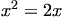, where saying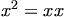.
HSN contribute: Help the site grow!

Looking for a Maths tutor in West Lothian? Just PM me!

### #24x_Claire_x

Site Swot

•• Members
•• 202 posts
• Location:Home =P
• Interests:Just Genreally Acting Like A Fool =P
• Gender:Female

Posted 27 April 2006 - 03:45 PM

lol i wish i knew what you guys are talking aboutJust Be Yourself It Doesn't Matter If It's Good Enough For Someone Else

• Location:Aboyne
• Gender:Male

Posted 03 May 2006 - 04:31 PM

I think instead of trying to find a specific fault in the working, it can be disproved anouther way.

If you have x=2x then you can say 0=2x-x so 0=x. Now if x is equal to zero then the original equation
(x^2 = x+x+x...) is irrelevant. Isnt it?

### #26kyle

Child Prodigy

•• Members
•• 841 posts
• Location:The dark side of the moon
• Gender:Male

Posted 03 May 2006 - 04:44 PM

Holy shit... the gift, do you remeber a year or so back when you played the Aboyne band night?

I'm the drummer from Silver Bullet, we played alongside you!You guys don't still play darkness stuff and wear spandex do you?• Location:Aboyne
• Gender:Male

Posted 03 May 2006 - 05:05 PM

lol cheers for bringing that up in the middle of a maths forum.......

Lol naa we keep the Darkness stuff to band practice. Its pretty much all our own stuff now. Spandex still occaisionally creeps in thoughHavent heard much from u guys recently, what u up to?

U doin higher maths? U know the proof? Or did u just see my name there? lol

### #28Richard_05

Child Prodigy

•• Members
•• 637 posts
• Interests:I'm not going to sit and type my grades, nor my plans for the future, nor a random fact. :)
• Gender:Male

Posted 03 May 2006 - 05:36 PM

I felt smart after reading this o.o

• Location:Aboyne
• Gender:Male

Posted 04 May 2006 - 03:12 PM

Ive been thinkin a lot about this and i dont think my last suggestion really disproves it.

However i think i can now disprove it properly.

It all starts in the second line, the biggest clue being in the statement (x lots of d/dx(x)). The problem is that the two x's, the one being differentiated and the other one, are the same x, the same variable, so if one is differentiated the other has to be as well.

This would give us (d/dx(x) lots of d/dx(x)) intsead.

In the next line we would then get (1+1+...+1) plus anouther (1+1+...+1)
which then gives us (x + x) which is 2x.

So d/dx( x) = 2x
so 2x=2x
so 2=2, 1=1 etc

I think ad_absurdum was the first one who suggested this when he said that x.(d/dx(x))d/dx( x)
I had the same idea, and carried it through, which in the end proves that 1 does indeed equal 1.

### #30George

Child Prodigy

••• 720 posts
• Location:West Lothian
• Gender:Male

Posted 04 May 2006 - 03:41 PM

This would give us (d/dx(x) lots of d/dx(x)) intsead.

In the next line we would then get (1+1+...+1) plus anouther (1+1+...+1)

Surely "d/dx(x) lots of d/dx(x)" is "1 lot of 1" i.e. 1?

Differentiation doesn't work like that anyway - e.g. for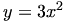, or "3 lots of x2", we don't do "d/dx(3) lots of d/dx(x2)" - that would mean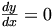because we have 0 lots of d/dx(x2).

I'm convinced the problem lies with the "x lots of" though### #31theos

• Gender:Male

Posted 07 May 2006 - 04:45 PM

I agree with thegift_guitar. I hope my solution is helpfull if not rightYou are quite right george differentiation does not work by differentiating a constant however this is not what has been done here, as x is the variable which is being differentiatied repectively. This means it has to be differentiated in order to maintain consistency as one of the x variables changes the other one changes by the same degree so must be taken account of, as differentiation is basically a measurement for rate of change.

Basically you would have (x+x+x+x...) x lots of times

this can be differentiated by using the product rule.

The product rule gives us a method to differentiate the product of two or more functions.

It states that when k (x) = f (x) g (x)
then k ' (x) = f ' (x) g (x) + f (x) g ' (x)

f(x) = (x+x+x+x...) and g(x)= x

so (1+1+1+1...) xlots of times + (x+x+x+x...) 1 lots of times

this can be rewritten as x+ x = 2x

Also as

dy/dx(x²) = 2x

it shows that 2x = 2x ie 1 = 1

### #32TempName123

Fully Fledged Genius

•• Members
•• 1,155 posts
• Gender:Male
• Location:...a womb
• Interests:mm.... to lazy to say

(and the womb bit works in the forums where it says "from" but not here duh just makes me sound.. well... weird!)
• Gender:Male

Posted 07 May 2006 - 04:48 PM

its in the maths textbook. the sign changes when u divide though.

Sorry, but I don't agree (or see where that would happen)!

no seriously there are about four of them in the Adv higher textbook.

QUOTE
x=y => x^2 = y^2 = xy
=> x^2 - xy = x^2 - y^2
=> x(x-y) = (x-y)(x+y)
=> x = x + y
=> x = 2x [since x=y]
=> 1 = 2

lifted straight form the textbook.

### #33Steve

Top of the Class

••• 435 posts
• Location:Edinburgh
• Gender:Male

Posted 07 May 2006 - 04:54 PM

no seriously there are about four of them in the Adv higher textbook.

Yes, but that's not the problem here - the error in your example is division by zero.

If you differentiate the expression properly then there is no contradiction.
HSN contribute: Help the site grow!

Looking for a Maths tutor in West Lothian? Just PM me!

### #34George

Child Prodigy

••• 720 posts
• Location:West Lothian
• Gender:Male

Posted 07 May 2006 - 06:21 PM

so (1+1+1+1...) xlots of times + (x+x+x+x...) 1 lots of times

That's an interesting way to think of it, but I don't think the product rule really applies in the way you've used it.

What we've really got is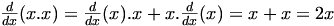using the product rule.

I think everyone seems to at least be agreeing that the problem is with the "x lots of", and it being ignored when differentiating.

• Location:Aboyne
• Gender:Male

Posted 08 May 2006 - 05:19 PM

Theos, thanks for agreeeing. Your working of those steps is more precise than mine. The product rule was what i was following but i didnt name it because i dont think strictly speaking it is higher level maths.

George, i think what you have worked through is the same as what theos is saying, the product does indeed give us 2x and so now both sides of the equation are equal to 2x. Therefore 2=2 etc...

### #36George

Child Prodigy

••• 720 posts
• Location:West Lothian
• Gender:Male

Posted 08 May 2006 - 06:28 PM

George, i think what you have worked through is the same as what theos is saying, the product does indeed give us 2x and so now both sides of the equation are equal to 2x. Therefore 2=2 etc...

My point was that the product rule doesn't work in the way that theos used it.

f(x) = (x+x+x+x...) and g(x)= x

That implies that you are differentiating x(x+x+x+x...), which isn't what we're after. If you still think it's right to use the product rule, can you explain what the "..." in f(x) means?

I think the 'proof', and the discussion of it, is really showing how tricky it can be to deal with expressions involving "...". If you don't have your wits about you, they can trip you up!

• Location:Aboyne
• Gender:Male

Posted 08 May 2006 - 07:33 PM

Ok the "..." means that it is just continued, so what we really mean is X+X+X+X+X continued on untill we have added X together X number of times.

When you say we are differentiating x(x+x+x+x....) and this is not what we wont, you are wrong i think. This is what we want. Because we have x added to gether x number times then our two variables are both x. Its best not to think of it as x(x+x+x+x...) but rather as the two seperate equations f(x) and g(x), which we are trying to differntiate. f(x) is the (x+x+x+x...) and g(x) is the (x lots of). In this case the product rule does work exactly as theos described.

In your post earlier u used to the product rule to show that we get x+x = 2x. This correct, this is what me and theos have done aswell, so in your post you have done the same as us, im not sure why you think we are doing it differently because im pretty sure were not. Im sorry if this doesnt explain it, i know its not the best explanation. Theos might be able to help more......????

### #38George

Child Prodigy

••• 720 posts
• Location:West Lothian
• Gender:Male

Posted 08 May 2006 - 08:00 PM

OK, my point was really that f(x) = (x+x+x+x...) is "x lots of x", as you said yourself. This is just the same as the RHS in the original 'proof', which we want to differentiate.

Now, using the f(x) and g(x) theos had defined:
g(x)f(x) = x(x+x+x+x....) = x2+x2+... = x3
And that really isn't what we want to differentiate.

Another problem is, f(x) = (x+x+x+x...)f'(x) = 1+1+1+... = x is exactly the mistake that was made in the original 'proof'.

So, using the product rule on x.x is OK, but trying to use it with anything involving "..." leads to trouble.

### #39Mr H

Site Swot

•• Members
•• 156 posts
• Location:Soonagonner Retirement Home
• Gender:Male

Posted 08 May 2006 - 08:19 PM

I'm glad that no-one has asked me for the answer!H tends 2 infinity

---------------------------------
Never argue with an idiot. They drag you down to their level then beat you with experience.

• Location:Aboyne
• Gender:Male

Posted 09 May 2006 - 03:12 PM

Ok please, for once and for all, ENLIGHTEN US!

#### 1 user(s) are reading this topic

0 members, 1 guests, 0 anonymous users